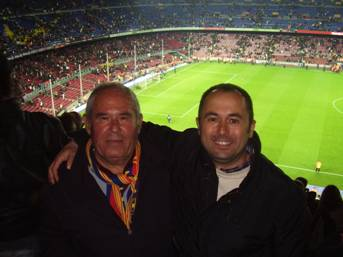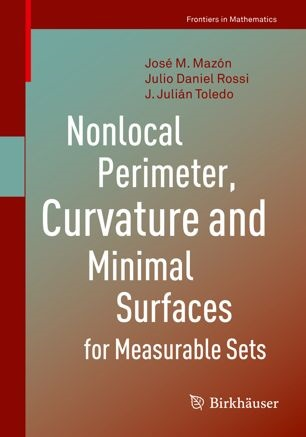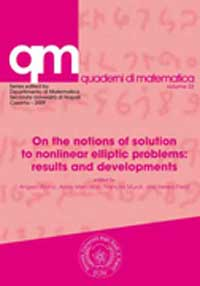Julián ToledoJulián Toledo & Julián Toledo

Edificio de Investigación, despacho 3.14
Dr. Moliner 50
46100 Burjassot
Spain

Docencia:
ver http://www.uv.es/anamat

Publications (Research articles/Books/Other publications)

Research articles

$$\blacksquare$$ Cahn-Hilliard equations on random walk spaces
Analysis and Applications, Vol. 21, No. 4 (2023) 959-1000.
https:///dx.doi.org/10.1142/S0219530523500045
J. M. Mazón and J. Toledo

$$\blacksquare$$ Nonlocal doubly nonlinear diffusion problems with nonlinear boundary conditions
J. Evol. Equ. (2023) 23:24, 83 pp.
https://doi.org/10.1007/s00028-022-00854-y
M. Solera and J. Toledo

$$\blacksquare$$ $$(BV,L^p)$$-decomposition, $$p=1,2$$ , of functions in Metric Random Walk Spaces
Adv. Calc. Var. 15 (2022), no. 3, 515–550.
https://doi.org/10.1515/acv-2020-0011
J. M. Mazón, M. Solera and J. Toledo

$$\blacksquare$$ Evolution problems of Leray-Lions type with nonhomogeneous Neumann boundary conditions in metric random walk spaces
Nonlinear Analysis (2020) 111813, 38 pp.
https://doi.org/10.1016/j.na.2020.111813
J. M. Mazón, M. Solera and J. Toledo

$$\blacksquare$$ The total variation flow in metric random walk spaces
Calc. Var. 59, 29 (2020), 64 pp.
https://doi.org/10.1007/s00526-019-1684-z
J. M. Mazón, M. Solera and J. Toledo

$$\blacksquare$$ The heat flow on metric random walk spaces
J. Math. Anal. Appl. 483 (2020) 123645, 53 pp.
https://doi.org/10.1016/j.jmaa.2019.123645
J. M. Mazón, M. Solera and J. Toledo

$$\blacksquare$$ Nonlocal perimeter, curvature and minimal surfaces for measurable sets
Journal d'Analyse Mathématique 138 (2019), 235-279.
https://doi.org/doi:%2010.1007/s11854-019-0027-5
J. M. Mazón, J. D. Rossi and J.Toledo

$$\blacksquare$$ Optimal mass transportation for costs given by Finsler distances via $$p$$-Laplacian approximations
Adv. Calc. Var. 2018; 11(1): 1-28.
https://doi.org/10.1515/acv-2015-0052
J. M. Mazón, N. Igbida, J. D. Rossi and J. Toledo

$$\blacksquare$$ An optimal matching problem with constrains
Rev Mat Complut (2018) 31: 407-447.
https://doi.org/10.1007/s13163-018-0256-7
J. M Mazón, J. D. Rossi and J. Toledo

$$\blacksquare$$ On the uniqueness and numerical approximations for a matching problem
SIAM J. OPTIM. 27 (2017) 2459-2480.
https://doi.org/10.1137/16M1105001
N. Igbida, V. T. Nguyen and J. Toledo

$$\blacksquare$$ The Heat Content for Nonlocal Diffusion with Non-singular Kernels
Advanced Nonlinear Studies 17 (2017), 255-268.
https://doi.org/10.1515/ans-2017-0005
J. M. Mazón, J. D. Rossi and J. Toledo

$$\blacksquare$$ Fractional $$p$$- Laplacian evolution equations (click)
J. Math. Pures Appl. 105 (2016), 810-844.
https://doi.org/10.1016/j.matpur.2016.02.004
J. M. Mazón, J. D. Rossi and J. Toledo

$$\blacksquare$$ A nonlocal $$1$$-Laplacian and median values (click)
Publ. Mat. 60 (2016), 27-53.
https://doi.org/10.5565/PUBLMAT_60116_02
J. M. Mazón, M. Pérez-Llanos, J. D. Rossi and J. Toledo

$$\blacksquare$$ Optimal mass transport on metric graphs (click)
SIAM J. Optim. 28 (2015), 1609-1632
https://doi.org/10.1137/140995611
J. M. Mazón, J. D. Rossi and J. Toledo

$$\blacksquare$$ Optimal matching problems with costs given by Finsler distances (click)
Communications on Pure and Applied Analysis 14 (2015), 229-244.
https://doi.org/10.3934/cpaa.2015.14.229
J. M. Mazón, J. D. Rossi and J. Toledo

$$\blacksquare$$ On optimal matching measures for matching problems related to the Euclidean distance (click)
Mathematica Bohemica 139 (2014), 553-566.
https://dml.cz/handle/10338.dmlcz/144132
J. M. Mazón, J. D. Rossi and J. Toledo

$$\blacksquare$$ Mass transport problems obtained as limits of $$p$$-Laplacian type problems with spatial dependence (click)
Adv. Nonlinear Anal. 3 (2014), 133-140.
https://doi.org/10.1515/anona-2013-0022
J. M. Mazón, J. D. Rossi and J. Toledo

$$\blacksquare$$ Mass transport problems for the Euclidean distance obtained as limits of $$p$$-Laplacian type problems with obstacles (click)
J Differential Equations 256 (2014), 3208-3244.
https://doi.org/10.1016/j.jde.2014.01.039
J. M. Mazón, J. D. Rossi and J. Toledo

$$\blacksquare$$ An optimal matching problem for the Euclidean distance (click)
SIAM J. Math. Anal. 46 (2014), 233-255.
https://doi.org/10.1137/120901465
J. M. Mazón J. D. Rossi and J. Toledo

$$\blacksquare$$ An optimal transportation problem with a cost given by the Euclidean distance plus import/export taxes on the boundary (click)
Rev. Mat. Iberoam. 30 (2014), no. 1, 277-308.
https://doi.org/10.4171/rmi/778
J. M. Mazón, J. D. Rossi and J. Toledo

$$\blacksquare$$ On the best Lipschitz extension problem for a discrete distance and the discrete infinity-Laplacian (click)
J. Math. Pures Appl. (9) 97 (2012), no. 2, 98-119.
J. M. Mazón, J. D. Rossi and J. Toledo

$$\blacksquare$$ A Monge-Kantorovich mass transport problem for a discrete distance (click)
J. Funct. Anal. 260 (2011), 3494–3534.
N. Igbida, J. M. Mazón, J. D. Rossi and J. Toledo

$$\blacksquare$$ Local and nonlocal weighted $$p$$-laplacian evolution equations with Neumann boundary conditions (click)
Publ. Mat. 55 (2011), 27-66.
F. Andreu, J. M. Mazón, J. D. Rossi and J. Toledo

$$\blacksquare$$ Degenerate elliptic equations with nonlinear boundary conditions and measures data (click)
Ann. Sc. Norm. Super. Pisa Cl. Sci. (5) 8 (2009), no. 4, 767–803.
Andreu, Fuensanta; Igbida, Noureddine; Mazón, José M.; Toledo, Julián

$$\blacksquare$$ The limit as $$p\to ∞$$ in a nonlocal $$p$$-Laplacian evolution equation: a nonlocal approximation of a model for sandpiles (click)
Calc. Var. Partial Differential Equations 35 (2009), no. 3, 279–316.
Andreu, F.; Mazón, J. M.; Rossi, J. D.; Toledo, J.

$$\blacksquare$$ A nonlocal $$p$$-Laplacian evolution equation with nonhomogeneous Dirichlet boundary conditions (click)
SIAM J. Math. Anal. 40 (2008/09), no. 5, 1815–1851.
Andreu, F.; Mazón, J. M.; Rossi, J. D.; Toledo, J.

$$\blacksquare$$ Obstacle problems for degenerate elliptic equations with nonhomogeneous nonlinear boundary conditions (click)
Math. Models Methods Appl. Sci. 18 (2008), no. 11, 1869–1893.
Andreu, Fuensanta; Igbida, Noureddine; Mazón, José M.; Toledo, Julián

$$\blacksquare$$ A nonlocal $$p$$-Laplacian evolution equation with Neumann boundary conditions (click)
J. Math. Pures Appl. (9) 90(2008), no. 2, 201–227.
Andreu, F.; Mazón, J. M.; Rossi, J. D.; Toledo, J.

$$\blacksquare$$ Renormalized solutions for degenerate elliptic-parabolic problems with nonlinear dynamical boundary conditions and $$L^1$$-data (click)
J. Differential Equations 244 (2008), no. 11, 2764–2803.
Andreu, F.; Igbida, N.; Mazón, J. M.; Toledo, J.

$$\blacksquare$$ The Neumann problem for nonlocal nonlinear diffusion equations (click)
J. Evol. Equ. 8 (2008), no. 1,189–215.
Andreu, Fuensanta; Mazón, José M.; Rossi, Julio D.; Toledo, Julián

$$\blacksquare$$ $$L^1$$ existence and uniqueness results for quasi-linear elliptic equations with nonlinear boundary conditions (click)
Ann. Inst. H. Poincaré Anal. Non Linéaire 24 (2007), no. 1, 61–89.
Andreu, F.; Igbida, N.; Mazón, J. M.; Toledo, J.

$$\blacksquare$$ Quasi-linear elliptic problems in $$L^1$$ with non homogeneous boundary conditions (click)
Rend. Mat. Appl. (7) 26 (2006), no. 3-4, 291–314.
Ammar, K.; Andreu, F.; Toledo, J.

$$\blacksquare$$ A degenerate elliptic-parabolic problem with nonlinear dynamical boundary conditions (click)
Interfaces Free Bound. 8 (2006),no. 4, 447–479.
Andreu, F.; Mazón, J. M.; Toledo, J.; Igbida, N.

$$\blacksquare$$ Quasi-linear diffusion equations with gradient terms and $$L^1$$ data (click)
Nonlinear Anal. 56 (2004), no. 8, 1175–1209.
Andreu, F.; Segura de León, S.; Toledo, J.

$$\blacksquare$$ Blow-up for a class of nonlinear parabolic problems (click)
Asymptot. Anal. 29 (2002), no. 2, 143–155.
Andreu, F.; Mazón, J. M.; Simondon, F.; Toledo, J.

$$\blacksquare$$ Porous medium equation with absorption and a nonlinear boundary condition (click)
Nonlinear Anal. 49 (2002), no. 4, Ser. A: Theory Methods, 541–563.
Andreu, F.; Mazón, J. M.; Toledo, J.; Rossi, J. D.

$$\blacksquare$$ Regularity for entropy solutions of parabolic $$p$$-Laplacian type equations (click)
Publ. Mat. 43 (1999), no. 2, 665–683.
Segura de León, S.; Toledo, J.

$$\blacksquare$$ Global existence for a degenerate nonlinear diffusion problem with nonlinear gradient term and source (click)
Math. Ann.314 (1999), no. 4, 703–728.
Andreu, F.; Mazón, J. M.; Simondon, F.; Toledo, J.

$$\blacksquare$$ Existence and uniqueness for a degenerate parabolic equation with $$L^1$$-data (click)
Trans. Amer. Math. Soc. 351 (1999), no. 1, 285–306.
Andreu, F.; Mazón, J. M.; Segura de León, S.; Toledo, J.

$$\blacksquare$$ Asymptotic behaviour of solutions of quasi-linear parabolic equations with nonlinear flux (click)
Comput. Appl. Math. 17 (1998),no. 2, 201–215.
Andreu, F.; Mazón, J. M.; Toledo, J.

$$\blacksquare$$ Attractor for a degenerate nonlinear diffusion problem with nonlinear boundary condition (click)
J. Dynam. Differential Equations 10 (1998), no. 3, 347–377.
Andreu, F.; Mazón, J. M.; Simondon, F.; Toledo, J.

$$\blacksquare$$ Quasi-linear elliptic and parabolic equations in $$L^1$$ with nonlinear boundary conditions (click)
Adv. Math. Sci. Appl. 7(1997), no. 1, 183–213.
Andreu, F.; Mazón, J. M.; Segura de León, S.; Toledo, J.

$$\blacksquare$$ Large-time behavior of solutions of a system of PDE's governing diffusion processes in a heterogeneous medium (click)
Differential Integral Equations 10 (1997), no. 1, 165–180.
Mazón, José M.; Toledo, Julián

$$\blacksquare$$ Stabilization of solutions of the filtration equation in $$\mathbb{R}^N$$ (click)
Houston J. Math. 22 (1996), no. 1, 183–198.
Mazón, J. M.; Toledo, J.

$$\blacksquare$$ Stabilization of solutions of the filtration equation with absorption and non-linear flux (click)
NoDEA Nonlinear Differential Equations Appl. 2 (1995), no. 3, 267–289.
Andreu, F.; Mazón, J. M.; Toledo, J.

$$\blacksquare$$ Asymptotic behaviour of solutions of the filtration equation in bounded domains
Dynam. Systems Appl. 3 (1994), no. 2, 275–295.
Mazón, José M.; Toledo, Julián

Books and Chapters

$$\blacksquare$$ Variational and Diffusion Problems in Random Walk SpacesSeries:Progress in Nonlinear Differential Equations and Their Applications, Vol. 103, Birkhäuser, 2023.
José M. Mazón, Marcos Solera Diana, J. Julián Toledo-Melero

$$\blacksquare$$ Nonlocal Perimeter, Curvature and Minimal Surfaces for Measurable SetsSeries:Frontiers in Mathematics, Birkhäuser, 2019
Mazón, José M., Rossi Julio D., Toledo, J. Julián
https://www.springer.com/gb/book/9783030062422

$$\blacksquare$$ Nonlocal Diffusion Problems (click)Mathematical Surveys and Monographs, vol. 165. AMS, 2010.
Fuensanta Andreu-Vaillo, José M. Mazón, Julio D. Rossi, and J. Toledo-Melero

$$\blacksquare$$ Degenerate Elliptic Equations with Nonlinear Boundary ConditionsChapter in On the notions of solution to nonlinear elliptic problems: results and developments. pp 67-104. (click)
Quaderni di matematica, vol. 23 (2009).
Edited by A. Alvino, A. Mercaldo, F. Murat and I. Peral.
F. Andreu, N. Igbida, J. M. Mazón and J. Toledo

Proceedings and surveys

$$\blacksquare$$ Gradient flows in metric random walk spaces
SeMA J. 79 (2022), no. 1, 3–35.
https://doi.org/10.1007/s40324-021-00272-z
J. M. Mazón, M. Solera and J. Toledo

$$\blacksquare$$ On Nonlinear Nonlocal Diffusion Problems (Survey)
International Journal of Biomathematics and Biostatistics 1 (2010), no. 3, 191-202.
J. M. Mazón, J. D. Rossi and J. Toledo

$$\blacksquare$$ Existence and uniqueness results for quasi-linear elliptic and parabolic equations with nonlinear boundary conditions
Free boundary problems, 11–21, Internat. Ser. Numer. Math., 154, Birkhäuser, Basel, 2007.
Andreu, F.; Igbida, N.; Mazón, J. M.; Toledo, J.

$$\blacksquare$$ Attractor for a degenerate nonlinear parabolic equation with a nonlinear flux
Proceedings of the IV Catalan Days of Applied Mathematics (Tarragona, 1998), 1–7, Univ. Rovira Virgili, Tarragona, 1998.
Andreu, F.; Mazon, J. M.; Toledo, J.; Simondon, F.

$$\blacksquare$$ Entropy solutions for a degenerated parabolic equation
Proceedings of the 3rd Catalan Days on Applied Mathematics (Lleida, 1996), 151–161, Univ. Lleida, Lleida.
Andreu, F.; Mazon, J. M.; Segura, S.; Toledo, J.

$$\blacksquare$$ Els inicis de la matemàtica moderna (Science outreach)
Chapter in ELS ORIGENS.
Monografies de les Seccions de Ciències, 11 (1994).
Universitat Catalana d’Estiu.
Institut d’Estudis Catalans.
J. Julià Toledo

Talks

A conference on Non Local Problems

A conference on Optimal Mass Transport Problems from $$p$$-Laplacian type Problems (Workshop on nonlinear equations, University Carlos III, Madrid, 2013)

A conference on Limit cases of nonlocal problems with Dirichlet boundary conditions (5th Iberian Mathematical Meeting, University of Aveiro, 2014)

5ème Ecole de Printemps EDP Non-Linéaire. Mathématiques et Interactions: modèles non locaux et applications.
Ecole Supérieure de Technologie d'Essaouira, du 27 au 30 Avril 2015.
http://www.este.ucam.ac.ma/EPEDPNL2015/Ecole
Courses by:
Jérôme Coville
Abderrahim El Moataz Billah
Grzegorz Karch
Julián Toledo (click)

A conference on A PDE approach to optimal matching problems (VI jornadas de Análisis Matemático, University of Alicante, 2018)

Conferences

Remembering Fuensanta Andreu
A conference in memoriam: http://www.uv.es/edpnol/fuensanta/
http://www.ams.org/mathscinet-getitem?mr=2512556

Nonlinear Partial Differential Equations
A congress on the occasion of J.M. Mazón's 60th birthday
Burjassot (Valencia) from 01/07/2013 to 05/07/2013.

Conference: Partial Differential Equations in Valencia
http://edpnol.blogs.uv.es/
Colleagues web pages

$$\int_\Omega f$$